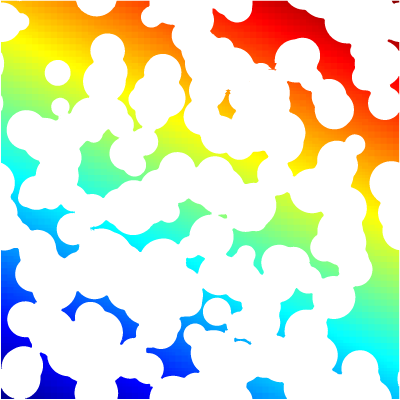# Hydrostatic balance with refined embedded boundaries

This test case is related to flow in a complex porous medium. We check that for a “closed pore” medium, hydrostatic balance can be recovered. This is not trivial in particular when the spatial resolution is variable, since pressure values need to be interpolated (at least) to second-order close to embedded boundaries. This behaviour depends on the restriction and prolongation operators used close to embedded boundaries.

#include "embed.h"
#include "navier-stokes/centered.h"
#include "view.h"

We use a similar porous medium as in porous.c but with enough disks so that the pores are entirely closed.

void porous (scalar cs, face vector fs)
{
int ns = 200;
double xc[ns], yc[ns], R[ns];
srand (0);
double a = 0.5, b = 0.04;
for (int i = 0; i < ns; i++)
xc[i] = a*noise(), yc[i] = a*noise(), R[i] = 0.02 + b*fabs(noise());

vertex scalar phi[];
foreach_vertex() {
phi[] = HUGE;
for (double xp = -L0; xp <= L0; xp += L0)
for (double yp = -L0; yp <= L0; yp += L0)
for (int i = 0; i < ns; i++)
phi[] = intersection (phi[], (sq(x + xp - xc[i]) +
sq(y + yp - yc[i]) - sq(R[i])));
}
fractions (phi, cs, fs);
fractions_cleanup (cs, fs);
}

int main()
{
origin (-0.5, -0.5);

init_grid (1 << 6);

The events of the Navier-Stokes solver are called “by hand”.

  event ("metric");
event ("defaults");

porous (cs, fs);
#if 0
refine (level < 8 && cs[] > 0 && cs[] < 1);
porous (cs, fs);
#else
porous (cs, fs);
porous (cs, fs);
porous (cs, fs);
#endif

const face vector G[] = {1.,2.};
a = G;

The system is quite stiff.

  TOLERANCE = 1e-6;
NITERMAX = 100;
mgp.nrelax = 100;
alpha = fm;
dt = 1.;

event ("acceleration");
#if 1
event ("projection");
#else
foreach()
p[] = G.x[]*x + G.y[]*y; // exact pressure
foreach_face()
uf.x[] -= alpha.x[] ? dt*alpha.x[]*face_gradient_x (p, 0) : 0.;

face vector gf[];
foreach_face()
gf.x[] = fm.x[] ? fm.x[]*a.x[] - alpha.x[]*(p[] - p[-1])/Delta : 0.;

trash ({g});
foreach()
foreach_dimension() {
g.x[] = (gf.x[] + gf.x)/(fm.x[] + fm.x + SEPS);
//      assert (fabs(g.x[]) < 1e-6);
}

correction (dt);
#endif

We check the convergence rate and the norms of the velocity field (which should be negligible).

  fprintf (stderr, "mgp %g %g %d %d\n", mgp.resb, mgp.resa, mgp.i, mgp.minlevel);
fprintf (stderr, "umax %g %g\n", normf(u.x).max, normf(u.y).max);

The pressure is hydrostatic, in each of the pores.Pressure field.

  view (fov = 19, width = 400, height = 400);
draw_vof ("cs", filled = -1, fc = {1,1,1});
}